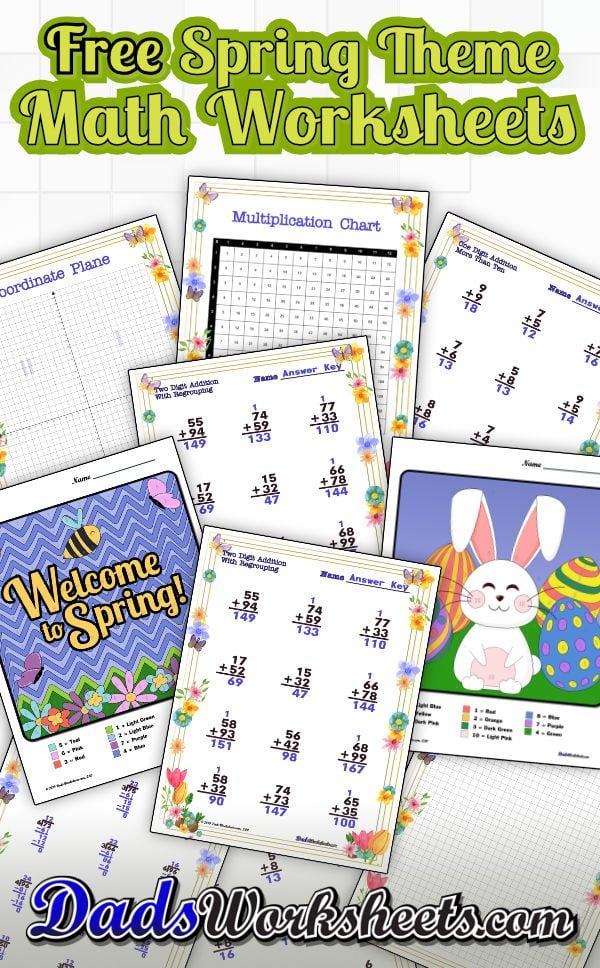Math Worksheets

# Spring Math Worksheets

What better time of year to start growing some new math skills than Spring! This page has a collection of color by number worksheets appropriate for kindergarten through fourth grade, covering addition, subtraction, multiplication and division operations. There is also a collection of simple spring math worksheets with fun spring flower themes, along with a multiplication chart, hundreds chart, graph paper and coordinate plane!

## Spring Flowers, Spring Showers and Spring Math Worksheets!

It's not just about flowers and bunny rabbits... If you're celebrating Spring, it can be a lot of fun to introduce some math practice into the season!

The worksheets on this page feature both math fact practice and color by number activities, including coloring pages that incorporate math facts for addition, subtraction, multiplication and division. Students solve basic bath fact problems, and use the answers to determine what color to apply to different areas of the worksheet.

These worksheets are appropriate for students in kindergarten through 4th grade. The color by number pages are great for kindergarten, and some of the addition worksheets can be introduced at this time as well. The addition and subtraction worksheets are appropriate for first grade and second grade students, while the multiplication and division worksheets more appropriate for third and 4th grade students.